Emergent Gravity/Non-linear U (1) Gauge Theory Correspondence | OMICS International
Journal of Astrophysics & Aerospace Technology

# Emergent Gravity/Non-linear U (1) Gauge Theory Correspondence

K Priyabrat Pandey, Abhishek K Singh, Sunita Singh and Supriya Kar*

Department of Physics & Astrophysics, University of Delhi, New Delhi-110 007, India

*Corresponding Author:
Supriya Kar
Department of Physics & Astrophysics
University of Delhi, New Delhi-110 007, India
Tel: +91 9911918174
E-mail: [email protected]

Received Date: September 17, 2013; Accepted Date: December 12, 2013; Published Date: December 19, 2013

Citation: Priyabrat Pandey K, Singh AK, Singh S, Kar S (2013) Emergent Gravity/ Non-linear U (1) Gauge Theory Correspondence. J Astrophys Aerospace Technol 2:101. doi:10.4172/2329-6542.1000.101

Copyright: © 2013 Priyabrat Pandey K, et al. This is an open-access article distributed under the terms of the Creative Commons Attribution License, which permits unrestricted use, distribution, and reproduction in any medium, provided the original author and source are credited.

Visit for more related articles at Journal of Astrophysics & Aerospace Technology

#### Abstract

We revisit a five dimensional Kaluza-Klein gravity, in presence of a cosmological constant, with a renewed interest in a type IIA string theory. An irreducible space-time curvature scalar K, leading to a non-perturbation theory of emergent quantum gravity, is addressed on a D4-brane underlying a two form gauge theory. An appropriate ansatz for a two form in the non-linear U (1) gauge theory is explored to obtain a nontrivial geometric torsion in a second order formalism. The quantum geometries, underlying torsion, in a low energy limit is shown to describe an AdS5 -brane black hole in Einstein vacuum. In the limit, we analyze the non-perturbation curvature K on S1 and argue for a plausible strong-weak coupling duality between a five dimensional non-perturbation curvature theory and the 4D perturbation non-linear gauge theory.

#### Keywords

Gravity; Quantum; Thermal; Temperature; Theory.

#### Introduction

An intrinsic notion of geometry, governing space-time curvature, is manifested beautifully in the success of the General Theory of Relativity (GTR). Importantly, the Einstein field equations confirm the presence of non-linear matter and establish a precise relation between the matter and geometry in GTR. With the help of an extra dimension to Einstein vacuum, the matter/geometry relation may be explored to view as a gauge/gravity duality or in particular AdS5-gravity/gauge theory duality established in literatures [1-3]. On the other hand, a pair of brane/antibrane may see to be contained within a higher dimensional brane in string theory [4-6]. Similar to AdS/CFT, an emergent gravity is believed to be projected along a preferred holographic direction in space, which possibly governs a cut-off scale on the boundary. Since a flat space is not associated with any holographic direction, it is promising to begin with Newtonian potential to describe the emergent gravity phenomenon as a non-relativistic limit of GTR. As a result, the holographic direction may be seen to be governed by a gradient of the scalar potential, which in turn describes an accelerated frame and establishes the notion of temperature . A five dimensional classical space-time curvature may become apparent from four dimensional thermal quantum geometry. In fact with an extra dimension to the 4D Einstein gravity, i.e. a five dimensional stringy space-time , needs a careful analysis and may enlighten us on some of the quantum gravity effects.

In the recent past, the gravity has been conjectured as an emergent phenomenon . In particular, the gravity has been argued to emerge at a macroscopic level due to the entropic force described by the matter field, which in turn results in a deformed geometry . In addition a construction leading to an emergent gravity in a quantum field theory or a gauge theory has been proposed . Interestingly, the near horizon black hole geometries have been explored on a BPS D-brane in type II superstring theories [11-27]. In the context, the external geometries on a D-brane sourced by a zero mode of a two form may be exploited further to address some of the non-external quantum black holes underlying generalized non-perturbation formalism. In fact, the stringy deformations leading to geometric causal patches have been described on a non BPS D-brane [28-32] underlying a non-linear U (1) gauge theory. The emergent quantum vacua on a brane undergo a tunneling phenomenon and lead to some of the plausible classical vacua established in Einstein gravity.

In the article, we address a non-perturbation theory of quantum gravity sourced by a two form flux in a nonlinear U (1) gauge theory on a D4-brane. In fact, a two form has been argued to generate a nonperturbative space-time curvature on a vacuum created pair of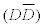3-brane at a Big Bang singularity [30,31]. The branes in a pair moved in opposite directions to each other. In section 2, we primarily outline a formalism leading to quantum gravity, underlying a geometric torsion, on a D4- brane in a type IIA superstring theory. In section 3, we construct an AdS5-brane Schwarzschild quantum vacuum sourced by the quantized flux of B4-field. In a low energy limit, the stringy deformations are ignored to obtain a classical AdS5 Schwarzschild black hole in five dimensions. In section 4, we consider the non-perturbation effective curvature on S1 and obtain a pair of U (1) gauge theories on a D3- brane and an anti D3-brane. A geometric torsion in five dimensions is argued to source the space-time curvatures on a pair of branes in a second order formalism. In a low energy limit, the torsion-dynamics may be ignored to describe a priori two different brane and anti-brane Universes. In the limit, a non- perturbation quantum theory of gravity in 5D is argued to be described by a weak coupling non-linear U (1) gauge theory on a D3-brane. We conclude by summarizing the article in section 4.

#### Emergent quantum gravity in 5D: A non-perturbation formalism

We begin with Kaluza-Klein gravity in presence of a cosmological constant Ʌ ≠ 0. The action is given by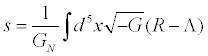(1)

Where GN denotes the (appropriate) Newton’s constant. Now, we revisit an open string effective metric derived on a D4-brane in type IIA superstring theory in ten dimensions . A constant Bmn is known to couple to a U (1) electromagnetic field Fmn to define a nonlinear field strength Fmn=Fmn+(2πα′)−1Bmn on a D-brane. Since the global modes in a two form cannot be gauged away, a non-linear field strength may as well be signified by a two form i.e. Fmn → Bmn. Then, an emergent metric on a D4-brane may be given by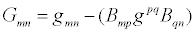(2)

Where non-dynamical gmn describes a flat line-element. The emergent metric on a D-brane is established in the folk lore of superstring theory. Interestingly, a nontrivial metric sourced by a nonlinear U (1) electric charge has been shown to govern various near horizon black hole geometries on a BPS brane [14-18]. They describe the external black holes on a D-brane. It may be noted that an emergent metric a priori may not incorporate all the local degrees of Einstein gravity except on a D3-brane. Nevertheless, it does not rule out the possibility of other forms of gravity on a Dp-brane presumably for p ≠ 3. In addition, a non-zero vacuum energy density sourced by a nonzero energy momentum tensor in the formalism may allow the emergent curvature theory of torsion to be compared with Einstein gravity in presence of a cosmological constant. Needless to mention that the cosmological constant is dynamical in Einstein gravity. For instance, it incorporates local degree(s) in BTZ black hole in three dimensions.

In the article, we address a generalized notion of emergent gravity on a D4-brane by exploiting the Poincare duality in the U (1) gauge theory on its five dimensional world-volume. The duality incorporates a dynamical two form in lieu of a one form on a D4-brane. It may be recalled that the mass-less spectrum in a closed string theory includes a Neveu-Schwartz two form source Bmn in the string world-sheet dynamics. The conformal invariance in the quantum world-sheet theory is known to describe an effective closed string action in ten dimensional space-time which includes a three form gauge curvature H3 in addition to Einstein gravity and a scalar dilation. Since closed strings are tangential to a D-brane, for a constant dilation a nontrivial curvature on a brane is controlled purely by a H3, which in turn may be identified with a gauge theoretic torsion in a non-linear U (1) gauge theory. Thus, the non-zero modes of two form induced on a D4-brane do play a significant role to incorporate a gauge theoretic curvature on its world-volume.

We shall see that an emergent space-time curvature on a D4-brane, in a generalized sense, may be described by a propagating geometric torsion H3, which may be viewed as a modified H3 in the formalism. Since closed strings, or Einstein gravity, decouples from a D-brane, the gravity turns out to be an emergent notion sourced exclusively by a two form flux in the formalism. In order to address a space-time curvature from a gauge theoretic, we exploit the two form gauge connections to appropriately modify the covariant derivative in a second order formalism. It is given by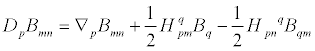(3)

Where a gauge connection (−2)Tmnp=Hmnp. Under an iteration H3 → H3, the geometric torsion in a second order formalism turns out to be non-perturbative. Alternately, it may be defined with all order corrections in B2 in a perturbation gauge theory. Formally, a geometric torsion may be expressed in terms of gauge theoretic torsion and its coupling to two form. It is given by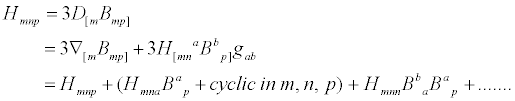(4)

It may seen to be uniquely fixed by Dpgmn=0. A priori, the U (1) gauge invariance under a two form transformation may seen to be broken in presence of higher order terms underlying a two form coupling to H3 in the gauge theory. Nevertheless under a two form transformation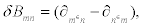the gauge invariance may seen to restored in the lorentz scalar H2 with an emerging metric fluctuation fmn in the formalism. It further re-assures the need for a second order formalism underlying a dynamical geometric torsion in a space-time curvature theory. The metric fluctuation  in the perturbation gauge theory may a priori be expressed as: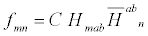(5)

Where C is an arbitrary constant and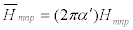. For an infinitesimal deformation, the metric fluctuation may be approximated to yield:(6)

An emergent metric fluctuation within a perturbation theory naturally transforms a gauge theoretic curvature to a geometric. Then, the effective metric (2) may see to incorporate the quantum fluctuation and may be re-expressed as: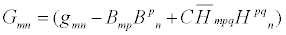(7)

Thus, an all order coupling to two form, or an exact perturbation gauge theory, may alternately be viewed as a non-perturbation curvature theory on a D4-brane. These two equivalent descriptions underlying a gauge theoretic and a geometric are remarkable in the formalism. Presumably they hint at a strong-weak coupling duality underlying a non-perturbation space-time curvature and a perturbation gauge theory on a D4-brane. We work out the commutator between the appropriate covariant derivatives in second order formalism underlying a geometric torsion [28-32]. For a Minkowskian line element, the commutator simplifies and is given by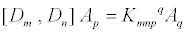Where the fourth order curvature tensor may be expressed as: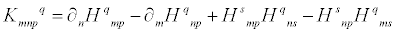(8)

An irreducible curvature scalar may be worked out to describe an effective space-time curvature theory on a D4-brane. Then, the action underlying a dynamical geometric torsion in a non-perturbation theory of quantum gravity may be given by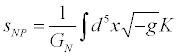(9)

Apparently, the effective curvature theory formally identifies with the Kaluza-Klein gravity. The cosmological constant in Kaluza- Klein action becomes redundant in an effective curvature dynamics sourced by a two form flux in superstring theory. However these two descriptions are completely independent of each other. In fact, effective curvature formalism underlies a non-perturbation theory of quantum gravity. The field equations may be given by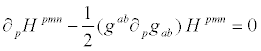(10)

In a low energy limit, the quantum gravity may see to reduce to Kaluza-Klein gravity in five dimensions. Most importantly, the generic curvature tensor Kmnpq modifies the Riemann-Christoffel curvature Rmnpq in Kaluza-Klein gravity. For a non-dynamical geometric torsion, the geometric curvature Kmnpq precisely reduces to Rmnpq and Ʌ ≠ 0. The fourth order curvature tensor is a generalized curvature obtained in a non-perturbation quantum theory of gravity on a D4-brane. In other words, the Riemannian notion of geometry leading to a typical Kaluza- Klein gravity is restored for a constant torsion. As a result, constant torsion geometry in the formalism is indistinguishable from the dynamical metric geometry.

#### AdS5 Schwarzschild black hole sourced by a two form

We consider an ansatz for a B-field to obtain a quantum AdS5 Schwarzschild black hole on a D4-brane. The B2-flux is given by: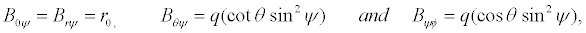(11)

Where 0 < θ ≤ π, 0 < ψ ≤ π, 0 ≤ φ ≤ 2π and (r0 and q) are arbitrary constants. The non-vanishing components of torsion become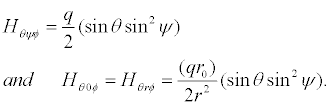(12)

The geodesic describing an AdS5 black hole may be obtained from the two-form using the relation (2) around AdS vacua. Interestingly, the obtained geometry may also be viewed via an appropriate Weyl scaling  of the emergent metric (7) obtained from a Minkowski line element on a D4-brane. In other words, a two form in a non-linear U (1) gauge theory on a D4-brane is exploited to create non-trivial spacetime on a pair of3-brane from a gauge theoretic vacuum at a Big Bang singularity [30,31]. The fifth dimension, transverse to a pair of3-brane, becomes large in a low energy limit. A brane is at an inaccessibly large time-like separation from its anti-brane and the effect of torsion becomes negligibly small in the limit. Then, the AdS5 classical black hole on a D4-brane in the regime may be approximated to yield: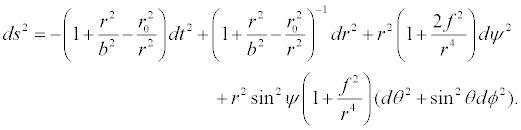(13)

Where f=q (cot θ sin ψ) and b denotes the AdS radius. The deformed S3 geometry in AdS5 black hole is a new feature in the formalism. Importantly, the deformation to the AdS5 Schwarzschild black hole in a typical Kaluza-Klein gravity is essentially due to a two form in the formalism. In other words, the broken S3-symmetry is restored for f=0, i.e. for a constant torsion and the AdS5 geometry (13) reduces to the AdS2×S3 Schwarzschild black hole geometry. In fact, the S3 deformation, by an S2, is independent of the torsion and is solely a characteristic of the non-vanishing two-form. Since a dynamical two form is dual to a scalar potential, the notion of temperature (T) may be seen to be governed by the deformation geometry in the formalism. For a constant torsion, the curvature is described by the Riemannian geometry and the deformation vanishes leading to T=0. It is thought provoking to believe that the association of temperature is possibly defined naturally in any formalism defined with a non-vanishing two-form. For instance, the deformed black hole geometry obtained on a non-commutative D3-brane [15,18], is a consequence of the noncommutative parameter and has been argued to be the origin of nonzero temperature .

#### Dual geometries: AdS5-brane ↔ U(1) gauge theory on a D3-brane

In this section, we outline a plausible string-weak coupling duality between a non-perturbative quantum theory of gravity in five dimensions and a perturbation non-linear electro-magnetic theory in four dimensions. The Kaluza-Klein compactification of the fifth dimension in the action (1) is worked out, a priori, to obtain the gravity and U (1) gauge curvatures. The generalized curvature K on S1 is obtained by Kaluza-Klein compactification of action (9) and is given by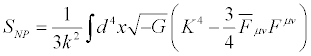(14)

Where the geometric scalar curvature K(4) is precisely governed by the torsion in the formalism. The fact that torsion is dual to an axion on an effective D3-brane ensures one degree of freedom. In addition, Fμν describes a geometric one form field with two local degrees on an effective D3-brane. A precise match among the (three) local degrees of torsion in K on S1 with that in K(4) and Fμν reassure the absence of a dynamical dilation field in the frame-work. The result is consistent with the fact that a two form on S1 does not generate a dilation field. The equation of motion for the one form is defined with an appropriate covariant derivative. It is given by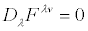(15)

Where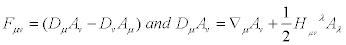. Apparently, one may obtain a U (1) field strength by incorporating a geometric torsion. A geometric electro-magnetic field may formally be expressed as: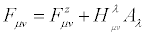(16)

It may appear that a torsion breaks the U (1) gauge invariance in Fμν in the formalism. Nevertheless, a two form U (1) gauge invariance leading to a metric fluctuation (5) reassures the gauge invariance in the lorentz scalar F2. Torsion, underlying the non-zero modes of a two form, incorporates non-linearity and presumably leads to a “fat” one form gauge field in the formalism. Thus, a geometric one form field is described by three local degrees on a D3-brane and would like to be governed by a non-linear U (1) gauge symmetry .

In other words, a two form in a non-linear U (1) gauge theory creates a pair of3-brane from a gauge theoretic vacuum. A generic space-time curvature, in four dimensions, emerges on a pair of brane at the Big Bang singularity [30,31]. Formally, the curvature scalars K(4) and F2 in equation (14) may randomly be assigned to a brane and an anti brane in a pair. Both the curvatures underlie torsion and hence they are dependent on each other. However for a large fifth dimension between a pair, the brane and anti-brane curvatures turns out to be independent to an observer on a brane-universe or on an anti brane- Universe. Thus from an observer perspective on a D3-brane-Universe, torsion may tend to disappear for an inaccessibly large time-like separation or an extra large dimension between a pair. In the limit, the emergent non-perturbative brane-Universe may be identified with a classical perturbation theory of gravity. Interestingly, the geometric field strength (1) may be approximated by a gauge theoretic F2 and hence retain an explicit U (1) gauge invariance. It implies that the nonzero modes of a two form leading to a propagating torsion on a D4- brane become insignificant in a classical regime. Nevertheless, the zero modes do play a vital role to govern a non-linear U (1) gauge symmetry on a D3-brane . Then, the irreducible space-time non-perturbative curvature (9) may alternately be viewed through a perturbation non linear gauge theory in a dual channel. Then, the irreducible perturbative dynamics in a classical regime takes a simple form and is given by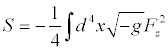(16)

The low energy non-linear U (1) gauge theory in 4D dual to a nonperturbative quantum gravity in 5D is remarkable. In a low energy limit, the stringy torsion effects are ignored to obtain a perturbative Kaluza-Klein gravity in five dimensions on a D4-brane. Our analysis firstly re-assures Einstein assertion that the space-time curvature is completely governed by the non-linear field. Secondly, the underlying duality is accompanied with a prior surprise due to its amazing low energy correspondence between the Kaluza-Klein gravity in 5D and a non-linear U (1) gauge theory in 4D. Interestingly, the gravity/gauge theory correspondence is along the line of the holographic idea.

On the other hand, the apparent duality between the Kaluza-Klein gravity and gauge theory may be better understood in a closed string theory. For instance, we consider a D=10 type IIB string on S1×K3 obtained by one of the author in a collaboration . The NS-NS sector in 5D may be worked out for a consistent truncation to yield Kaluza- Klein gravity (9). In particular, the geometric scalar curvature in the formalism may be identified with the gauge curvature in string theory, i.e. K ≡−(dB)2, in a dual channel with a covariant constant dynamical two-form. In fact, the obtained AdS5 geometry (13) may be viewed as an underlying AdS5×S5 geometry in type IIB string theory. This in turn, corresponds to the open string boundary gauge theory on a D3-brane . In other words, the non-linear U (1) gauge theory (17) may be identified with the D3-brane Born-Infield dynamics in string theory . As a result, the gravity/gauge theory correspondence may be viewed as closed/open string duality. The identification of gravity/ gauge theory correspondence underlying a non-perturbation theory of gravity and a non-linear gauge theory is unique to an emergent gravity on a D4-brane sourced purely by a two form in the formalism.

#### Concluding Remarks

A non-perturbation theory of quantum gravity, sourced by a two form flux, has been shown to emerge from a gauge theoretic vacuum on a D4-brane in a type IIA string theory. In particular, the emergent space-time curvature in a five dimensional quantum regime is shown to describe the dynamics of a geometric torsion in a second order formalism. In a low energy limit, the geometric torsion turns out to be non-propagating. Interestingly, the non-perturbation curvature reduces to the Riemannian in the limit. As a result, a non-perturbation quantum theory was shown to describe a five dimensional Einstein gravity in presence of a cosmological constant. It was argued that the non-zero modes of a two form become insignificant in the low energy limit though it’s zero modes source a cosmological constant.

A two form ansatz leading to a nontrivial torsion in five dimensions was shown to describe an AdS5 quantum black hole on a D4-brane. The space-time curvature on a brane was argued to be created from a gauge theoretic vacuum by a two form flux. In a low energy limit, the stringy deformations decouple from the quantum vacuum to yield a Schwarzschild AdS5 classical black hole on a brane as well as on an antibrane. The classical vacua identify themselves under r → −r and they are described by Einstein gravity in five dimensions.

On the other hand, the non-perturbation curvature on S1 was worked out for the geometric curvatures underlying an additional U (1) gauge symmetry in four dimensions. As a result a D4-brane may be alternately be viewed as a pair of3-brane, where each brane/anti-brane is governed by a non-linear U (1) gauge theory. In a low energy limit, the extra dimension between a pair was argued to be large. The local degrees in torsion was ignored in the limit to describe a non-linear U (1) gauge theory on a single D3-brane. In the limit, a non-perturbation quantum brane-gravity in five dimensions has been argued to describe a weak coupling gauge theory in four dimensions on a D3-brane.

#### Acknowledgements

The work of S.K. is partly supported by a research project under Department of Science and Technology, New Delhi, Govt.of India. AKS and SS, respectively, acknowledge Council of Scientific and Industrial Research and University Grants Commission for their fellowship.

#### References

Select your language of interest to view the total content in your interested language

### Article Usage

• Total views: 12353
• [From(publication date):
April-2014 - Jan 28, 2020]
• Breakdown by view type
• HTML page views : 8563Can't read the image? click here to refresh